Reflection Symmetry

Reflection Symmetry and Line of Symmetry

In our day to day lives, we witness numerous objects that have symmetry. An object or a figure is said to be symmetrical if it can be divided into two halves that are exactly equal. The line that divides the object or the figure into two equal halves or congruent halves is called the line of symmetry or the Mirror Line. In reflection symmetry, the first half is a reflection or mirror image of the second half.

Figures or objects can have more than one line of symmetry along which the figures or objects can be symmetrically divided. If a shape has one or more lines of symmetry, the shape is said to have reflection symmetry.

Important aspects of Reflection Symmetry:

• 1. A figure or shape or an object can have one or more than one line of symmetry.

• 2. The direction of the line of symmetry is not fixed.

• 3. Both the halves are congruent and mirror images of each other.

• Consider the shape of a heart held vertically. We can divide the heart into two equal halves along the central vertical line. This line is its line of symmetry.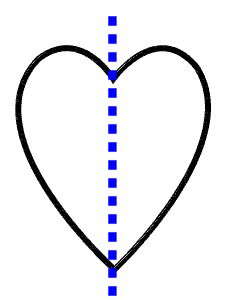The heart cannot be further folded to get the equal halves. Hence, the heart has only one line of reflection symmetry.

There are certain figures that possess more than one line of symmetry. E.g. – A rectangle: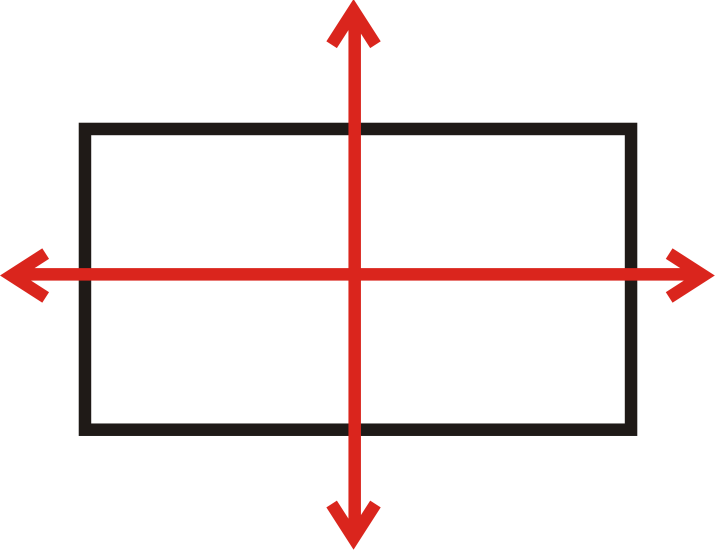As shown above, the rectangle can be divided into two equal halves either along the vertical line or along the horizontal line. Hence, a rectangle is said to have two axis of reflection symmetry.

One must note that not all shapes or figures have reflection symmetry. There are numerous shapes that cannot be divided into two congruent halves. Such shapes are called asymmetrical.

Consider a scalene triangle: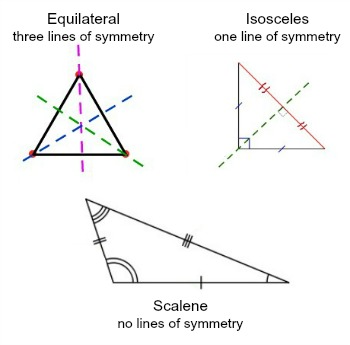This triangle cannot be divided into equal halves and hence does not have reflection symmetry.

Reflection symmetry can also be illustrated by everyday objects like given below: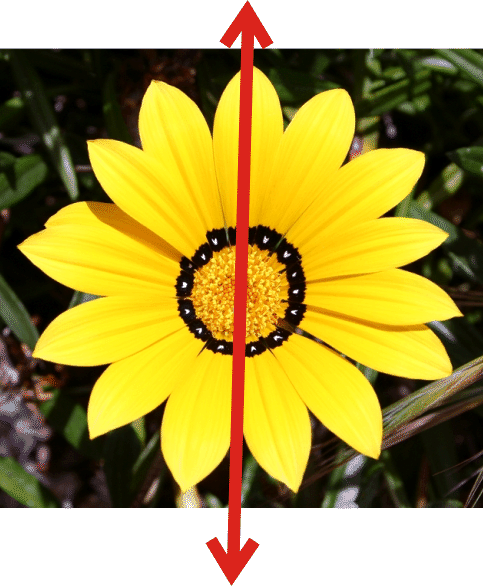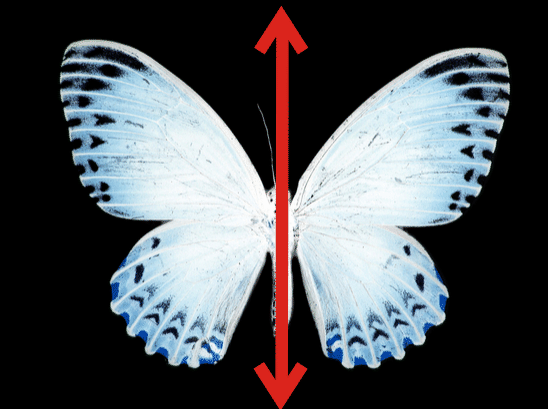Explain how many lines of reflection symmetry do circle, square and an equilateral triangle have?

Since a circle can be divided into equal halves along its diameter and the fact that infinite diameters can be drawn for a circle, a circle is said to have infinite lines of symmetry.

A square can be divided into equal halves by dividing horizontally, vertically or diagonally. Hence, a square is said to have 4 lines of symmetry.

Since the sides of an equilateral triangle are equal, it can be divided into equal halves by lines drawn from midpoint of a side joining the opposite vertex. So, an equilateral triangle has 3 lines of symmetry.

The isosceles triangle has two equal sides and therefore the line of symmetry or the reflection symmetry is two.

In all the above examples, there was only one line dividing the figure into two halves. There are many 3D objects where planes and not lines divide an object into two equal and congruent halves. Such planes are called planes of symmetry or planes of reflection. Consider a box or a container that you observe in a supermarket.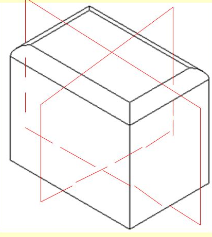Here, we can see that two planes divide the box into two equal halves. This object is said to have two planes of symmetry.

Reflection Symmetry is also called mirror symmetry or bilateral symmetry. In nature, reflection of mountains in rivers or lakes is a perfect example of reflection symmetry. Here, the line of symmetry is the ground line.Problems on Reflection Symmetry:
1. Give four examples of reflection symmetrical objects from your home or at school?
Answer: Refrigerator, Washing Machines, Television Sets, Blackboards.

2. Which is the following two lines dividing the object is the line of reflection symmetry?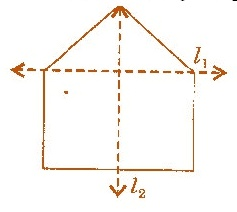Answer: The line l2 is the reflection symmetrical line because it divides the shape or figure into two equal halves. The two halves that we get on division by line l2 are mirror images of each other.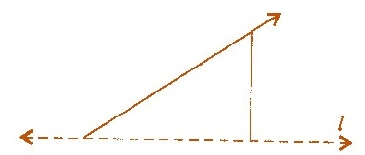3. Make the image above look symmetrical.

Answer: Consider that line l is the line of symmetry. Draw another ray starting at the base of the given line and it gives you two equal parts of the shape as shown below: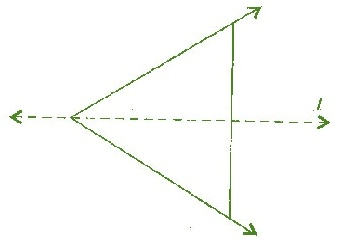The mirror image will complete the figure as L is the line of symmetry.

4. List the number of lines of symmetry in each of the shape given in the image below: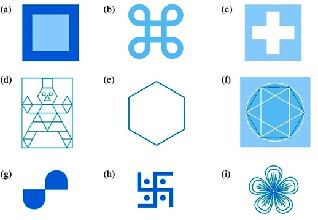Answer: (a) There are four lines of symmetry in this figure. (b) There are four lines of symmetry in this figure. (c) There are four lines of symmetry in this figure. (d) There are no lines of symmetry in this figure as the figure does not have any equal sides. (e) There are six lines of symmetry in this figure. (f) There are 6 lines of symmetry in this figure. (g) There are no lines of symmetry in this figure as it is sides are unequal. (h) There are no lines of symmetry in this figure. (i) There are three lines of symmetry in this figure.

5. Can you draw a triangle having only one line of Symmetry?

Answer: Yes, we can draw an Isosceles triangle as it has only one line of symmetry. The sides of an isosceles triangle are the same or equal.

6. Which triangle has three lines of Symmetry?

Answer: An Equilateral triangle has three lines of symmetry. All three sides of an equilateral triangle are the same.

7. How many lines of symmetry does a 5-pointed star have?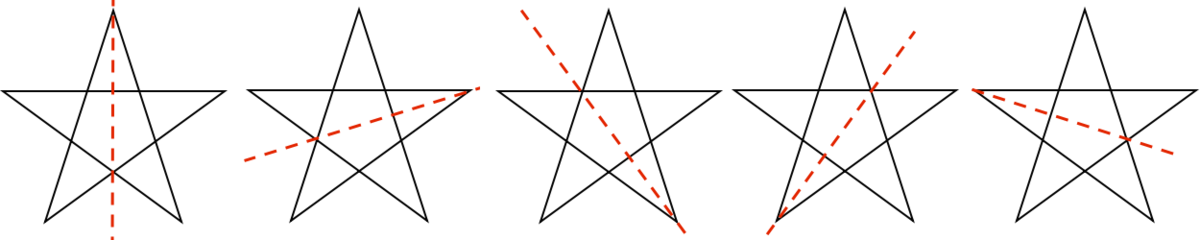Answer: As we can see, the regular star can be divided into 2 halves by 5 ways. Hence, this is said to have 5 lines of reflection symmetry.

8. Divide the Alphabets from A to Z on the basis of their line of symmetry that is vertical, horizontal, no line of symmetry and make a list of Lines of symmetry.
Answer: The alphabets A, H, I, M, O, T, U, V, W, X and Y have a vertical line of symmetry. Whereas, the alphabets B, C, D, E, H, I, K, O and X have a horizontal line of symmetry. Some alphabets such as F, G, J, N, P, Q, R, S and Z have zero or no line of symmetry.

9. Show that a human body has reflection symmetry.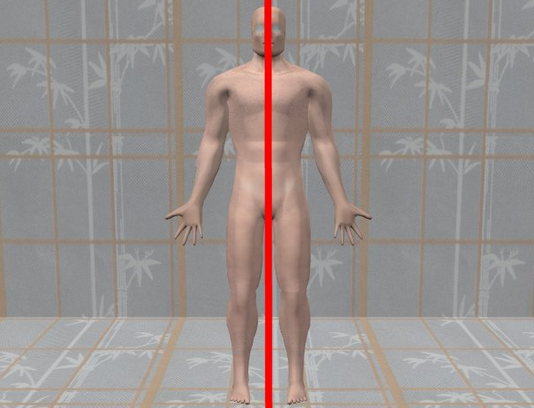Answer: When viewed from the outside, the human body seems to be symmetrical. A vertical axis of symmetry can be drawn through the center of the human body.

Knowledge of reflection symmetry plays an important role in calculation of moment of inertia of objects. This is a very important concept in structural civil designing.